Identities (square, cube of 2 complex numbers)

Chapter 5 Class 11 Complex Numbers
Concept wise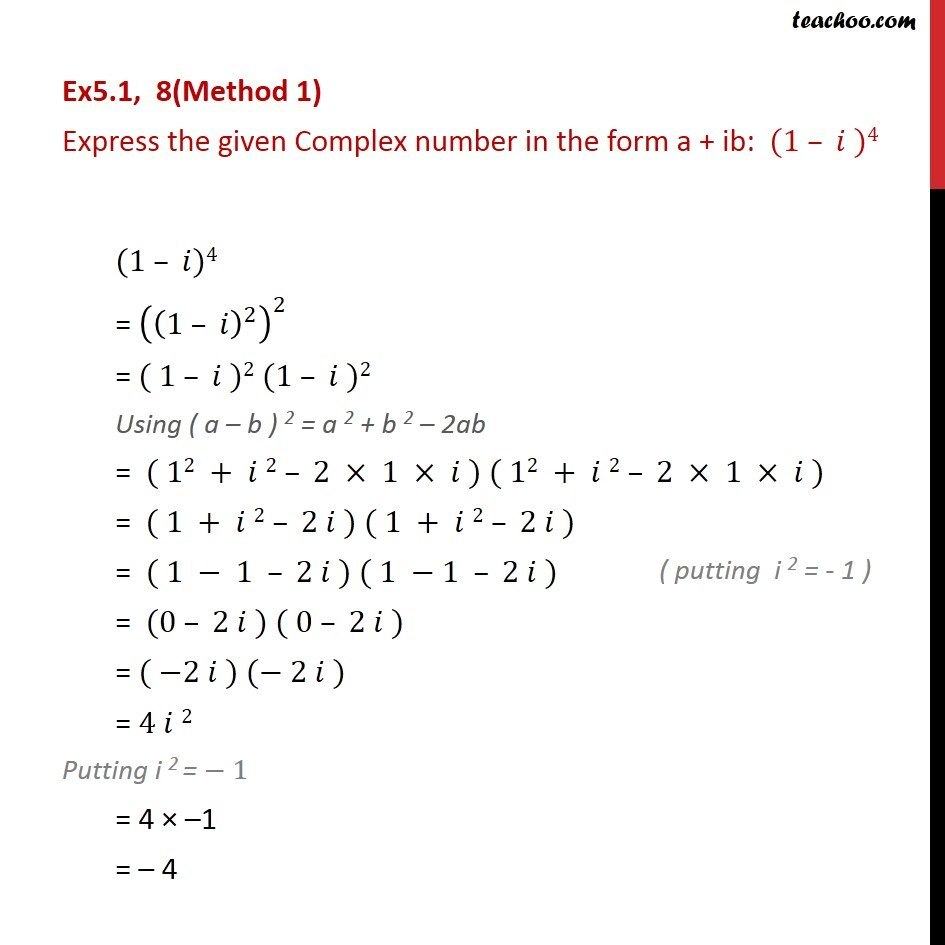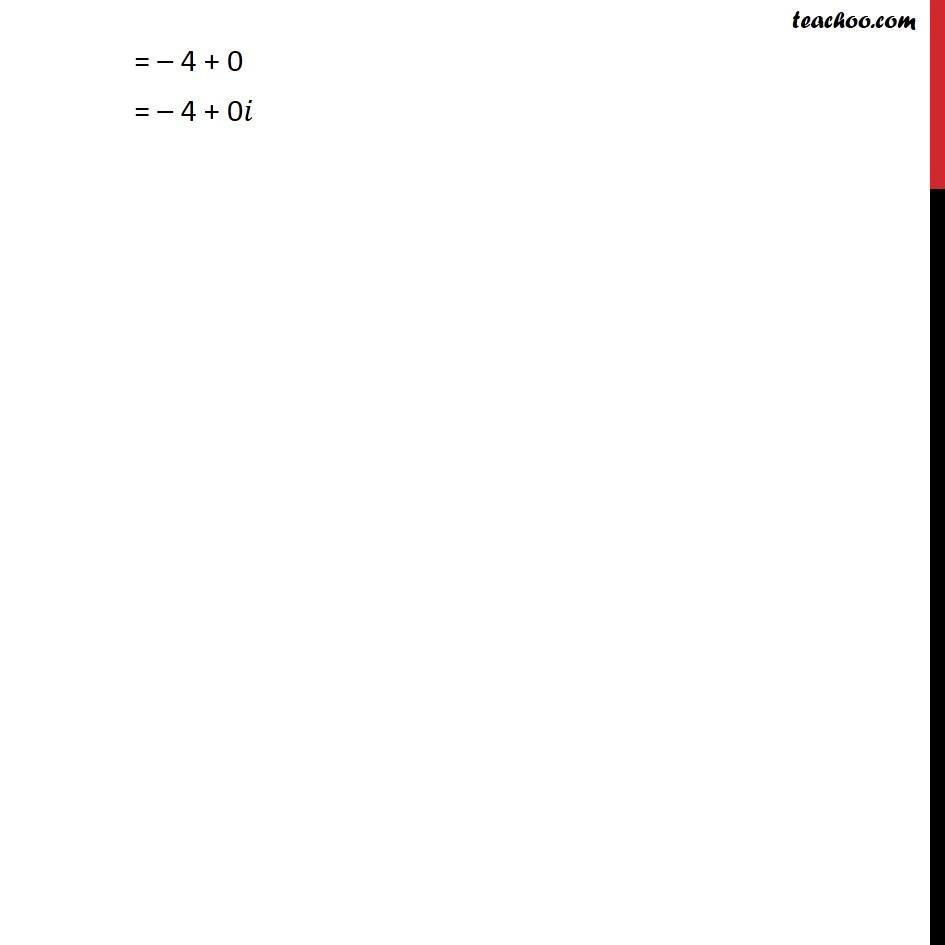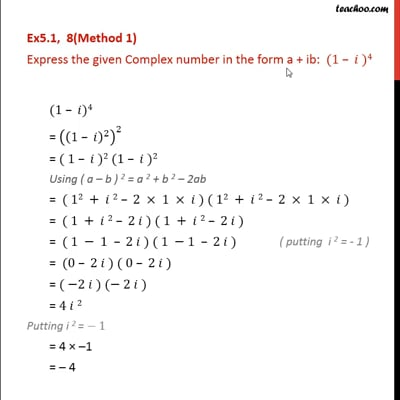This video is only available for Teachoo black users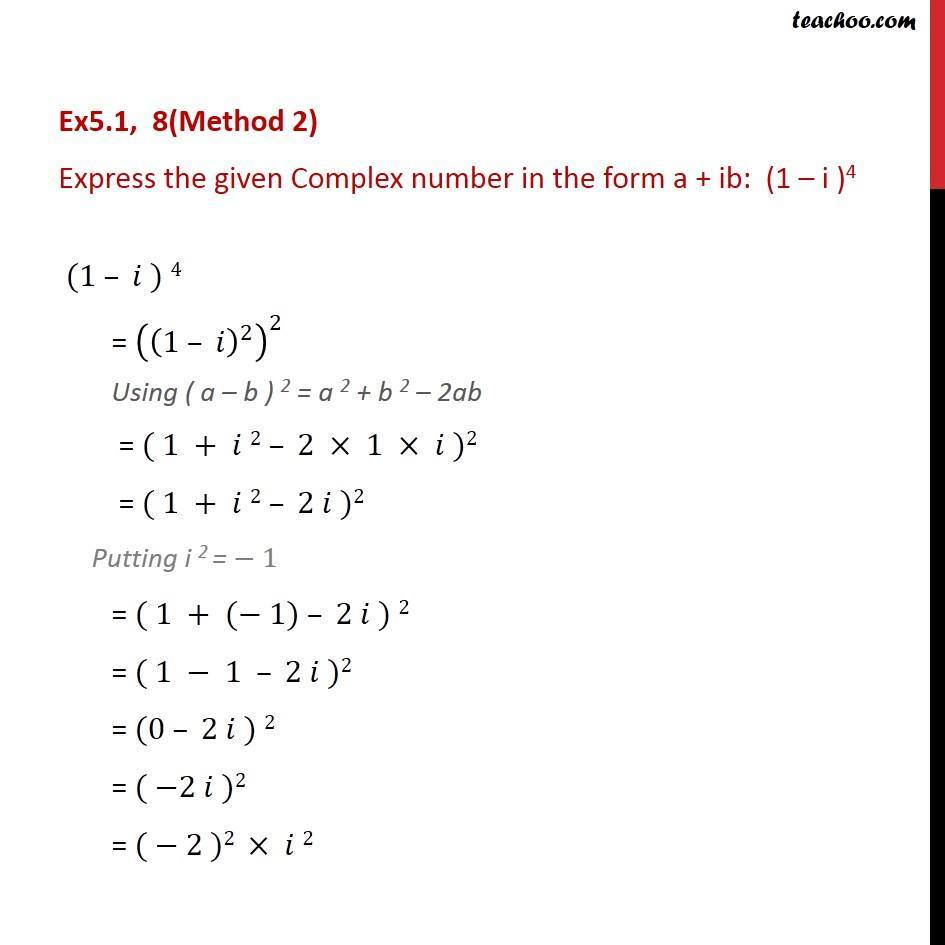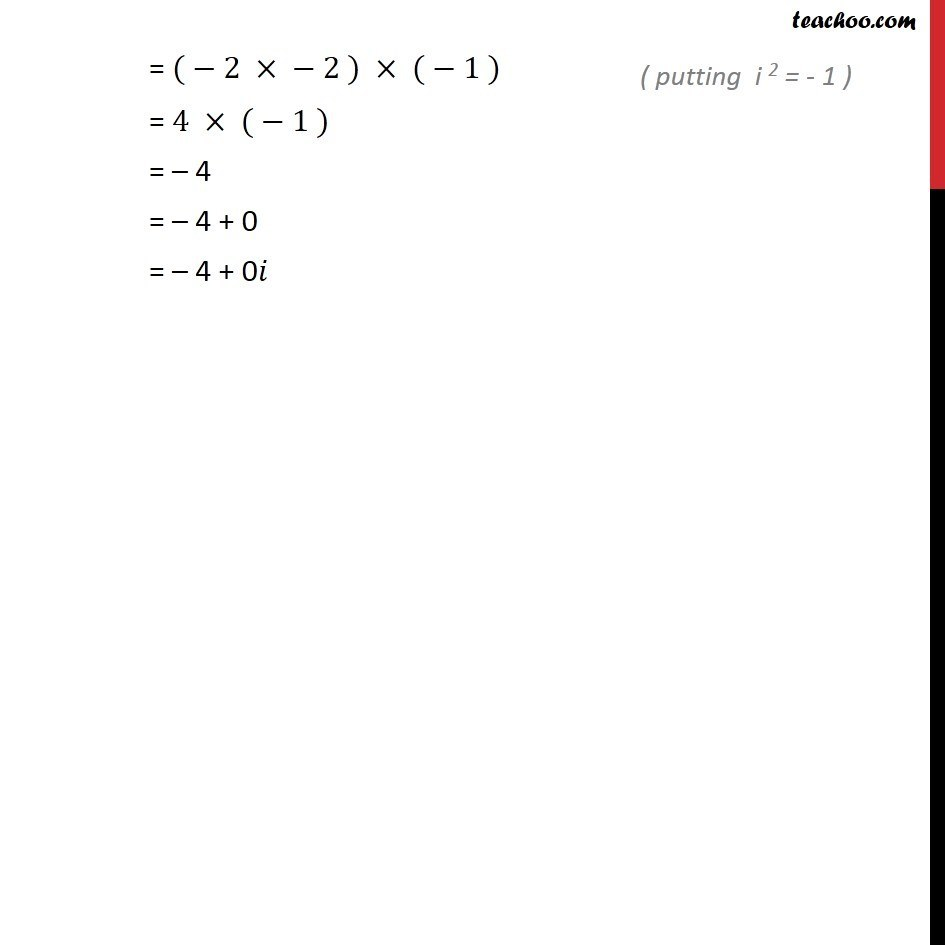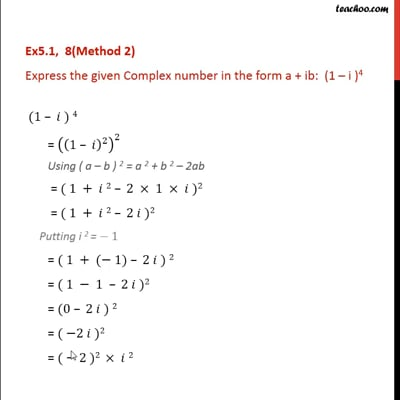This video is only available for Teachoo black users

Introducing your new favourite teacher - Teachoo Black, at only ₹83 per month

### Transcript

Ex5.1, 8(Method 1) Express the given Complex number in the form a + ib: (1 )4 (1 )4 = ((1 )^2 )^2 = ( 1 )2 (1 )2 Using ( a b ) 2 = a 2 + b 2 2ab = ( 12 + 2 2 1 ) ( 12 + 2 2 1 ) = ( 1 + 2 2 ) ( 1 + 2 2 ) = ( 1 1 2 ) ( 1 1 2 ) = (0 2 ) ( 0 2 ) = ( 2 ) ( 2 ) = 4 2 Putting i 2 = 1 = 4 1 = 4 = 4 + 0 = 4 + 0 Ex5.1, 8(Method 2) Express the given Complex number in the form a + ib: (1 i )4 (1 ) 4 = ((1 )^2 )^2 Using ( a b ) 2 = a 2 + b 2 2ab = ( 1 + 2 2 1 )2 = ( 1 + 2 2 )2 Putting i 2 = 1 "= "( 1 + ( 1) 2 ) 2 "=" ( 1 1 2 )2 "=" (0 2 ) 2 "=" ( 2 )2 "=" ( 2 )2 2 = ( 2 2 ) ( 1 ) = 4 ( 1 ) = 4 = 4 + 0 = 4 + 0